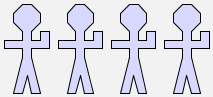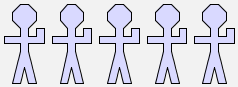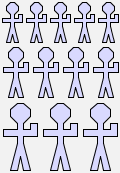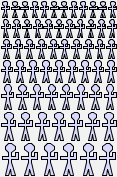# Worksheet 4: Recurrences and recursion

Suppose we want to draw rows of men of different lengths. It would be useful to have a function `manrow(n)` that, for any value of `n`, would give a row of `n` men. How can we define this function so that it works for any value of `n` .

For example `manrow(4)` would be a row of 4 men; let's call that picture `r4`:

 `define r4 = man \$ man \$ man \$ man`Similarly, let us call a row of five men `r5`:

 `define r5 =  man \$ man \$ man \$ man \$ man`What relates `r4` and `r5`? How can we go from a row of four men to a row of five men? We just need to add another man! So we can say:

`r5 = r4 \$ man`.

Similarly, we can say that `r4 = r3 \$ man` if `r3` is a row of three men.

Writing your answers in the same way, work out equations for `r3` and `r2`.

The expression `r1` refers to a row of just one man, which is the same as the expression `man`. Hence we should write the equation

`r1 = man`.

It would be ideal if we could define a function `manrow` that can take a number as an argument, and produce a row of men that is as long as that number. The way to do this is tricky to understand, so look at the definition below, and then read the explanation that follows it:

```define manrow(n) = manrow(n-1) \$ man when n > 1
| manrow(1) = man;
```

This definition of the function `manrow` consists of two equations, separated by a vertical bar character `|`. The first equation is used when `n > 1`, and states in general form our observations that `r4 = r3 \$ man`, and `r3 = r2 \$ man`, and so on. The other equation is simpler, and just restates the fact that `r1 = man` in terms of the function `manrow`.

You should enter this definition into GeomLab. On a British PC keyboard, the symbol `|` is entered by holding down the `Shift` key and pressing the `\` key, just to the left of the `Z`. At first sight, this equation appears to be defining the function `manrow` in terms of itself, and because of this, we call it a recursive definition. Despite the self-reference, the definition works because it shows us how to calculate, say, `manrow(5)` assuming we already know how to calculate `manrow(4)`, and applying the equation repeatedly can bring us down to the base case `manrow(1) = man`.

Having defined `manrow` as aboive, if you type an expression like manrow(5), then GeomLab expands the definition like this:

```manrow(5) = manrow(4) \$ man
= manrow(3) \$ man \$ man
= manrow(2) \$ man \$ man \$ man
= manrow(1) \$ man \$ man \$ man \$ man
= man \$ man \$ man \$ man \$ man.
```

The result is the image we expected:

 `manrow(5)`What does `manrow(4) & manrow(3) & manrow(2)` look like? Sketch the picture here:

 `manrow(4) & manrow(3) & manrow(2)`This picture looks a bit like a crowd, so let's try to define a function that can create crowds of different sizes.

To get a realistic-looking crowd, we will need to have rows that get larger in the number of men (and smaller in height) as we travel from bottom to top of the picture. The function `crowd(m, n)` can be defined so that `m` is the length of the bottom row in the picture, and `n` is the length of the top row. To save ourselves work, we can use the previously defined function `manrow` to draw the rows that we want.

If `m` and `n` are the same, then the crowd will consist of just one row:

```crowd(m, m) = manrow(m)
```

Otherwise, we are considering an expression of the form `crowd(m, n)`, where `m < n`. This crowd consists of a top row containing `n` men, and below it a smaller crowd with rows ranging from `m` to `n-1` men:

```crowd(m, n) = manrow(n) & crowd(m, n-1)
```

We can put these two equations together to make a recursive definition of the function `crowd`:

```define crowd(m, n) = manrow(n) & crowd(m, n-1) when m < n
| crowd(m, m) = manrow(m)
```

Here are two example images produced using our `crowd` function:

 `crowd(3, 5)``crowd(6, 12)`Try it, by putting the definitions of both `manrow` and `crowd` together one with one of these expressions, all in the same text.

This sheet has introduced an important new idea: that we can take a recurrence relation that describes a sequence of pictures, and turn it into the definition of a recursive function that generates pictures in the sequence. Simple recursive definitions have two parts: a rule that generates an element of the sequence from the previous element, and a starting rule that defines the first element. The idea of defining functions recursively is important, because it opens the possibility that a finite program can generate an infinite variety of behaviour by varying the argument that is passed to the function.

With a non-recursive function, each time the function is referred to, the formula that is the body of the function is used just once. With a recursive function, substituting the body of the function for a use of it may leave a formula that still contains a use of the function, so the definition may be used many times in computing the value of the function. In a wider setting, it is recursion that lets us use functions to model, understand, and compute with complex processes.